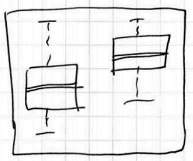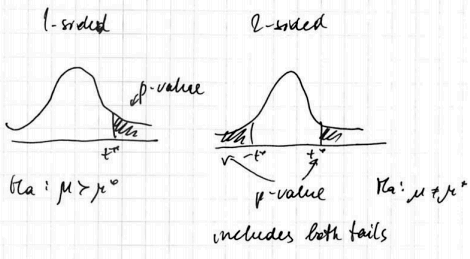# ML Wiki

## Hypothesis Testing

Hypothesis Testing is a framework of testing some assumptions in Inferential Statistics

• a result is statistically significant if it's very unlikely to have happened due to chance alone

### Motivation

Suppose we have 2 groups and we observe the difference between themQuestion:

• Is this difference significant?
• Could the difference be due to natural variability of the Sampling Distribution?
• Or we have something stronger?

Statistical tests (often as well called "Hypothesis tests" or "Tests of Significance") answer these questions.

## Structure of Statistical Test

### Summary

1. Determine the $H_0$ and $H_A$
2. Collect data and calculate a test statistic
3. Calculate the $p$-value
4. Make a conclusion based on it and on the context

### Step 1: Null and Alternative Hypotheses

• Formulate a hypothesis what you want to test and specify its alternative

Court case analogy: Innocent until proven guilty

• "Innocent" part
• null-Hypothesis $H_0$ (read as "H-naught")
• it's a skeptical position or position of no difference
• example: no relationships, no difference, etc
• we assume it's true
• "Guilty" part:
• alternative hypothesis $H_A$ (or $H_a$ or $H_1$)
• it's a new perspective
• this is what a researcher wants to establish
• example: relationship, change, difference

And we question we ask is do we have enough evidence to rule out any difference from the $H_0$ that are just due to chance?

Like in a court, we conclude that $H_A$ is true if we have evidence against $H_0$.

Alternatives could be

• one-sided (greater than or less than)
• two-sided (not equal)

So the first step is

• clearly specify the null and alternative hypotheses

### Step 2: Evidence - Test Statistics

• The evidence is provided by our data
• We need to summarize the data into a test statistics: a numerical summary of the data.

A test statistic is made under assumption that $H_0$ is true

So the 2nd step is

• Collect the data and calculate a test statistic assuming $H_0$ is true

### Step 3: $P$-value

• Is the evidence (the test statistics) good enough to reject the $H_0$?

$p$-value

• helps us to answer this question: it transforms the test statistic into a probabilistic scale:
• it's a number between 0 and 1 that quantifiers the strength of evidence against the $H_0$
• formally, $p$-value is a conditional probability of
• observing data favorable to $H_A$ and to the current data set
• given $H_0$ is true

• Assuming $H_0$ is true, how likely it is to observe a test statistic of this magnitude just by chance?
• And the numerical answer is the $p$-value

The smaller the $p$-value the stronger the evidence against $H_0$

Note!

• $p$-value cannot be interpreted as how likely it is that the $H_0$ is true.
• $p$-value tells you how unlikely the observed value of the test statistics (and more extreme value) is if the $H_0$ was true.

So the 3rd step is

• determine how unlikely the test statistic is if the $H_0$ is true (or, calculate the $p$-value)

### Step 4: Verdict

Based on the $p$-value make a verdict:

• $p$-value is not small
• $\Rightarrow$ conclude that the data is consistent with the $H_0$
• $p$-value is small
• $\Rightarrow$ then we have sufficient evidence against $H_0$ to reject it in favor of $H_A$
• we say "we fail to reject $H_0$"

Strength of the evidence:

• $p < 0.001$ - very strong
• $0.001 \leqslant p < 0.01$ - strong
• $0.01 \leqslant p < 0.05$ - moderate
• $0.05 \leqslant p < 0.1$ - weak
• $p \geqslant 0.1$ - no evidence

The result is statistically significant if the evidence is strong.

The final step:

• make a conclusion based on the $p$-value and on the context of the problem (important!)

## Terms

• Critical Value
• Power of a test
• Significance level
• $p$-value
• Type I and II Errors ("Decision Errors")

### Decision Errors

Summary 
$H_0$ is true $H_0$ is false
Reject $H_0$ Type I error
False positive
Correct outcome
True positive
Fail to reject $H_0$ Correct outcome
True negative
Type II error
False negative

### Significance Level

• The significance level of a test gives a cut-off for how small is small for a $p$-value
• It's denoted by $\alpha$ and called "desired level of significance"
• $\alpha$ shows how the testing method would perform in repeated sampling
• If $H_0$ is true and you use $\alpha = 0.01$, and you carry out a test repeatedly, with the same size of a sample each time, you will reject $H_0$ 1% of the time, and not reject 99% of the time
• If $\alpha$ is too small, you may never reject $H_0$, even if the true value is very different from the $H_0$

Choosing $\alpha$

• traditionally, $\alpha=0.05$
• if making Type I Errors is dangerous, or especially costly, choose small $\alpha$
• in this case we want very strong evidence to support $H_A$ before rejecting $H_0$
• if Type II Errors are more costly, then take higher $\alpha$, e.g. $\alpha=0.1$
• here we're careful about failing to reject $H_0$ when it's false

### Robustness

A statistical test is robust if the p-value is approximately correct even if some conditions aren't fully satisfied

## One-Sided vs Two-Sided

Alternative hypotheses $H_A$ could be one-sided or two-sided

• if it's one-sided we look only at the corresponding tail of our Sampling Distribution
• otherwise we look at both tails

Consider the following one-sample $z$-test for means:

### One-Sided

• $H_0: \mu = \mu_0, H_A: \mu > \mu_0$
• $\mu_0$ is called the "null value" because we assume it under $H_0$
• i.e. we want to check if population mean is larger than some value
• under the Normal Model we calculate the $z$-score and corresponding $p$ value of the right tail
•Analogously, for

• $H_0: \mu = \mu_0, H_A: \mu < \mu_0$
• we calculate the $p$-value based on the left tail
•### Two-Sided

Two-Sided alternative hypotheses looks at both left and right tails. E.g.

• $H_0: \mu = \mu_0, H_A: \mu \ne \mu_0$
•• if this case, we reject $H_0$ if the test statistics gets under any of the shaded tails
• i.e. the $p$-value is (typically) twice bigger than for one-sided tests

### $p$-values

• Don't misinterpret $p$-values (see what p-values say and what don't)
• A $p$-value is a measure of the strength of the evidence - so don't forget to report it

### Data Collection

Data Collection matters

• Sample wisely:
• use randomization to avoid flaws and biases

### Two-Sided Tests

Always try to use 2-sided tests

• Unless you're really sure you need one direction
•• $p$-value for one-sided test is 0.5 of p-value of 2-sided

One-sided hypotheses are allowed only before seeing the data

• it's never good to change 2-sided to 1-sided after observing the data
• it can cause twice more Type I errors (False positives - i.e. rejecting $H_0$ when it's true)

### Practical Significance

Statistical significance $\neq$ practical significance

• the larger the $n$, the smaller $p$-value
• A large $p$-value doesn't necessarily mean that the $H_0$ is true, there might be not enough power to reject it.

Small $p$-values can occur (in order of significance:)

• by chance
• data collection is biased
• violations of the conditions
• $H_0$ is false (the last one! - so be more careful about those above!)

So

• If multiple tests are carried out, some are likely to be significant by chance alone
• If $\alpha = 0.05$ we expect significant results 5% of the time, even when the $H_0$ is true
• $\Rightarrow$ be suspicious if you see only a few significant results when many tests have been carried out

### Data Snooping

• The test results are not reliable if the statements of the hypotheses are suggested by data.
• This is called data snooping - So hypotheses should be specified before any data is collected

## Relationship with Confidence Intervals

Main Article: Confidence Intervals and Statistical Tests

Some hypothesis can be checked with Confidence Intervals

• e.g. if the null value (the value under $H_0$) is included in the CI, then $p$-value is greater than $\alpha$ and we fail to reject $H_0$